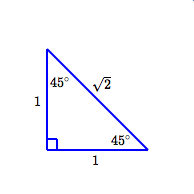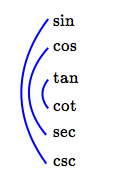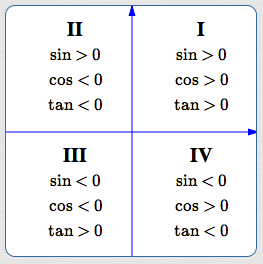﻿ Trigonometric Values of Special Angles

# TRIGONOMETRIC VALUES OF SPECIAL ANGLES

by Dr. Carol JVF Burns (website creator)
Follow along with the highlighted text while you listen!
Thanks for your support!
• PRACTICE (online exercises and printable worksheets)

With the work done in prior sections (particularly those listed below),
we have everything needed to efficiently find exact values for things like $\displaystyle\,\cos\frac{81\pi}{4}\,$ and $\,\csc (-2640^\circ)\,$.

All the necessary tools/ideas are repeated below, in-a-nutshell.
Having trouble following the brief discussion on this page?
If so, review the links above (in order)—they offer a much slower and kinder approach.

 two special triangles reciprocal relationships for trig functionsSOHCAHTOA Sine  Opposite  Hypotenuse  Cosine  Adjacent  Hypotenuse  Tangent  Opposite  Adjacent For example: $\displaystyle\csc = \frac{1}{\sin}$

 angle/number sine$\displaystyle\sin = \frac{\text{OPP}}{\text{HYP}}$ cosine$\displaystyle\cos = \frac{\text{ADJ}}{\text{HYP}}$ tangent$\displaystyle\tan = \frac{\text{OPP}}{\text{ADJ}}$ cotangent(reciprocalof tangent) secant(reciprocalof cosine) cosecant(reciprocalof sine) $0^\circ = 0 \text{ rad}$ $0$ $1$ $0$ not defined $1$ not defined $\displaystyle 30^\circ = \frac{\pi}{6} \text{ rad}$ $\displaystyle\frac 12$ $\displaystyle\frac{\sqrt 3}2$ $\displaystyle\frac1{\sqrt 3} = \frac{\sqrt 3}{3}$ $\sqrt 3$ $\displaystyle\frac{2}{\sqrt 3} = \frac{2\sqrt 3}{3}$ $2$ $\displaystyle 45^\circ = \frac{\pi}{4} \text{ rad}$ $\displaystyle\frac 1{\sqrt 2} = \frac{\sqrt 2}{2}$ $\displaystyle\frac 1{\sqrt 2} = \frac{\sqrt 2}{2}$ $1$ $1$ $\sqrt 2$ $\sqrt 2$ $\displaystyle 60^\circ = \frac{\pi}{3} \text{ rad}$ $\displaystyle\frac{\sqrt 3}{2}$ $\displaystyle \frac{1}{2}$ $\displaystyle \sqrt 3$ $\displaystyle\frac1{\sqrt 3} = \frac{\sqrt 3}{3}$ $2$ $\displaystyle\frac{2}{\sqrt 3} = \frac{2\sqrt 3}{3}$ $\displaystyle 90^\circ = \frac{\pi}{2} \text{ rad}$ $1$ $0$ not defined $0$($\cot := \frac{\cos}{\sin}$) not defined $1$Reciprocals retain the sign ($+/-$) of the original number. Therefore, in all the quadrants: Cosecant has the same sign as sine. Secant has the same sign as cosine. Cotangent has the same sign as tangent.

## Trigonometric Values for arbitrary special angles

In Special Triangles and Common Trigonometric Values, the ‘Locate–Shrink/Size–Signs’ method
was introduced for finding trigonometric values of special angles.

With additional tools and terminology now at hand, that discussion is presented more generally and efficiently here.

## the RRQSS (Reduce-Reference/Quadrant-Size/Sign) Technique

REDUCE:

[This step is optional.
If your angle isn't too big (say, $\,510^\circ\,$ or $\,-\frac{7\pi}{3}\,$),
then it may be easy for you to find its reference angle and quadrant, without ‘reducing’ it first.

As discussed in Reference Angles, remove any extra rotations from $\,\theta\,$:

 $\theta\,$ in DEGREES $\theta\,$ in RADIANS How many extra rotations (if any) in $\,\theta\,$? To answer this question, compute $\displaystyle\,n := \frac{|\theta|}{360^\circ}\,$, rounded to the nearest whole number. If $\,\theta\,$ is positive, then replace $\,\theta\,$ by $\,\theta - n\cdot 360^\circ\,$. If $\,\theta\,$ is negative, then replace $\,\theta\,$ by $\,\theta + n\cdot 360^\circ\,$. Now, your angle/number is manageable: it is between $\,-180^\circ\,$ and $\,180^\circ\,$. But, it has the same terminal point, so all the trigonometric values are the same! How many extra rotations (if any) in $\,\theta\,$? To answer this question, compute $\displaystyle\,n := \frac{|\theta|}{2\pi}\,$, rounded to the nearest whole number. If $\,\theta\,$ is positive, then replace $\,\theta\,$ by $\,\theta - n\cdot 2\pi\,$. If $\,\theta\,$ is negative, then replace $\,\theta\,$ by $\,\theta + n\cdot 2\pi\,$. Now, your angle/number is manageable: it is between $\,-\pi\,$ and $\,\pi\,$. But, it has the same terminal point, so all the trigonometric values are the same!

Lay off $\,\theta\,$ in the standard way:
• start at the positive $\,x\,$-axis
• positive angles are swept out in a counterclockwise direction; start by going up
• negative angles are swept out in a clockwise direction; start by going down
Determine the reference angle/number for $\,\theta\,$.
Determine the quadrant for $\,\theta\,$.
SIZE/SIGN:

Use the reference angle/number to find the correct SIZE of the desired trigonometric value.
Use the quadrant to find the correct SIGN of the desired trigonometric value.

## EXAMPLES

In this first example, the angles aren't too big, so the optional (reduce) step is skipped.

 Find: $\,\sec 510^\circ\,$ Find: $\displaystyle\,\cot (-\frac{7\pi}{3})\,$ REDUCE (skipped) [Dr. Burns would work with $\,510^\circ - 360^\circ = 150^\circ\,$.] (skipped) [Dr. Burns would work with $\,-\frac{7\pi}3 + \frac{6\pi}3 = -\frac{\pi}3\,$.] REFERENCE/QUADRANT$\,510^\circ\,$ is in quadrant II; the reference angle is $\,30^\circ\,$$\displaystyle\,-\frac{7\pi}{3}\,$ is in quadrant IV; the reference angle is $\,\displaystyle\frac{\pi}{3}\,$ SIZE/SIGN SIZE:   $\displaystyle\sec 30^\circ = \frac{2}{\sqrt 3}\,$ SIGN:   In quadrant II, the secant is negative. Thus: $\displaystyle\,\sec 510^\circ = -\frac{2}{\sqrt 3}\,$ SIZE:   $\displaystyle\cot\frac{\pi}{3} = \frac{1}{\sqrt 3}\,$ SIGN:   In quadrant IV, the cotangent is negative. Thus: $\displaystyle\,\cot(-\frac{7\pi}{3}) = -\frac{1}{\sqrt 3}\,$

In this final example, the angles are very big, so get rid of extra rotations (reduce) in the first step:

 Find: $\,\csc(-2640^\circ)\,$ Find: $\displaystyle\,\cos (\frac{81\pi}{4})\,$ REDUCE $\displaystyle \frac{|\theta|}{360^\circ} = \frac{2640^\circ}{360^\circ} \approx 7$ $-2640^\circ + 7\cdot 360^\circ = -120^\circ$ Work with $\,-120^\circ\,$ instead of $\,-2640^\circ\,$. $\displaystyle \frac{|\theta|}{2\pi} = \frac{81\pi/4}{2\pi} \approx 10$ $\displaystyle\frac{81\pi}{4} - 10\cdot 2\pi = \frac{81\pi}{4} - \frac{80\pi}{4} = \frac{\pi}4$ Work with $\displaystyle\,\frac{\pi}4\,$ instead of $\displaystyle\,\frac{81\pi}4\,$. REFERENCE/QUADRANT$\,-120^\circ\,$ is in quadrant III; the reference angle is $\,60^\circ\,$$\,\frac{\pi}4\,$ is in quadrant I; the reference angle is $\,\displaystyle\frac{\pi}{4}\,$ SIZE/SIGN SIZE:   $\displaystyle\csc 60^\circ = \frac{2}{\sqrt 3}\,$ SIGN:   In quadrant III, the cosecant is negative. Thus: $\displaystyle\,\csc(-2640^\circ) = -\frac{2}{\sqrt 3}\,$ SIZE:   $\displaystyle\cos\frac{\pi}{4} = \frac{1}{\sqrt 2}\,$ SIGN:   In quadrant I, the cosine is positive. Thus: $\displaystyle\,\cos\frac{81\pi}{4} = \frac{1}{\sqrt 2}\,$
Master the ideas from this section
by practicing the exercise at the bottom of this page.

When you're done practicing, move on to:
Fundamental Trigonometric Identities
On this exercise, you will not key in your answer.
However, you can check to see if your answer is correct.
PROBLEM TYPES:
 1 2 3 4 5 6 7 8 9 10 11 12 13 14 15 16 17 18 19 20 21 22
AVAILABLE MASTERED IN PROGRESS
 (MAX is 22; there are 22 different problem types.)